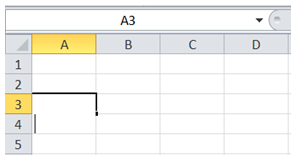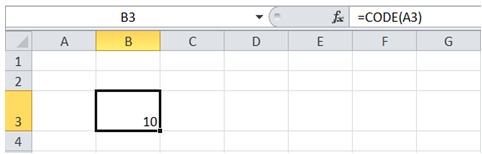## Non alphanumerical character code in Excel

For example, I want to enter a line break into a cell value using VBA. For that I would like to know the ASCII Character code for line break - how can I get the code from Excel?

To do it in Excel, here is the answer:

a) Click on a blank cell and then press "Alt + Enter" to enter a line break as shown below.b) To get the code for line break enter =CODE(A3) in a cell since line break has been introduced in cell A3. ASCII Value of line break is returned by the formula which can then be used in VBA.